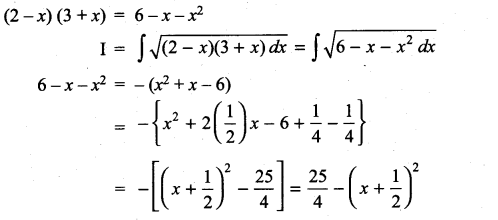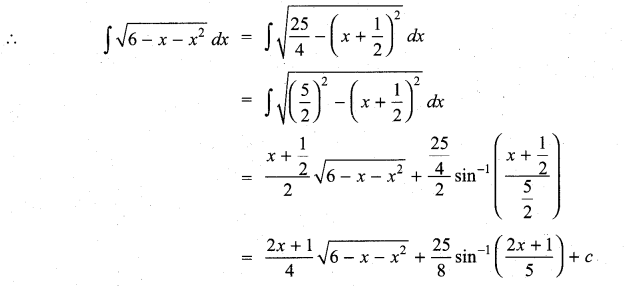# Samacheer Kalvi 11th Maths Solutions Chapter 11 Integral Calculus Ex 11.12

## Tamilnadu Samacheer Kalvi 11th Maths Solutions Chapter 11 Integral Calculus Ex 11.12

Integrate the following with respect to x:
Question 1.
(i) $$\sqrt{x^{2}+2 x+10}$$
Solution: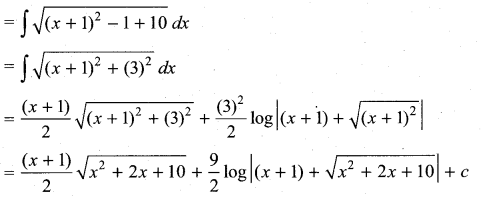(ii) $$\sqrt{x^{2}-2 x-3}$$
Solution: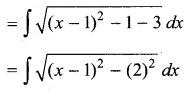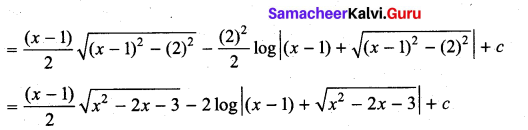(iii) $$\sqrt{(6-x)(x-4)}$$
Solution: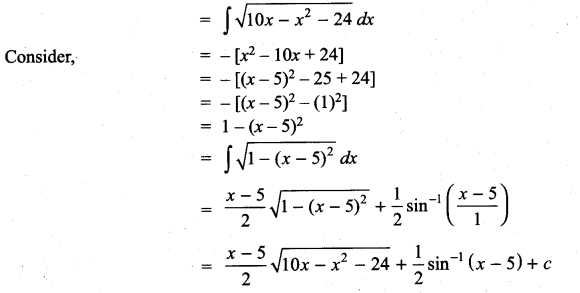Question 2.
(i) $$\sqrt{9-(2 x+5)^{2}}$$
Solution: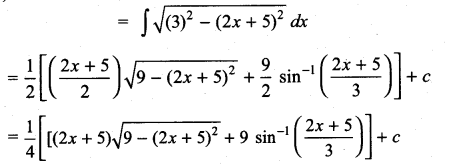(ii) $$\sqrt{81+(2 x+1)^{2}}$$
Solution: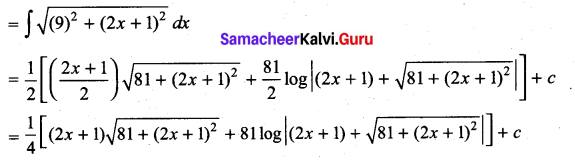(iii) $$\sqrt{(x+1)^{2}-4}$$
Solution: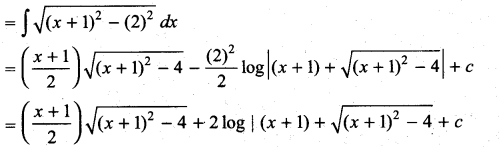### Samacheer Kalvi 11th Maths Solutions Chapter 11 Integral Calculus Ex 11.12 Additional Problems

Question 1.
$$\sqrt{(x+1)^{2}+4}$$
Solution: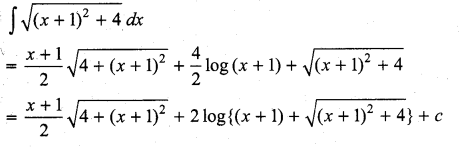Question 2.
$$\sqrt{(2 x+1)^{2}+9}$$
Solution: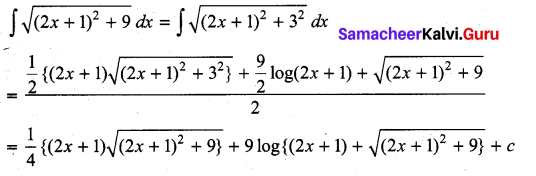Question 3.
$$\sqrt{x^{2}-3 x+10}$$
Solution: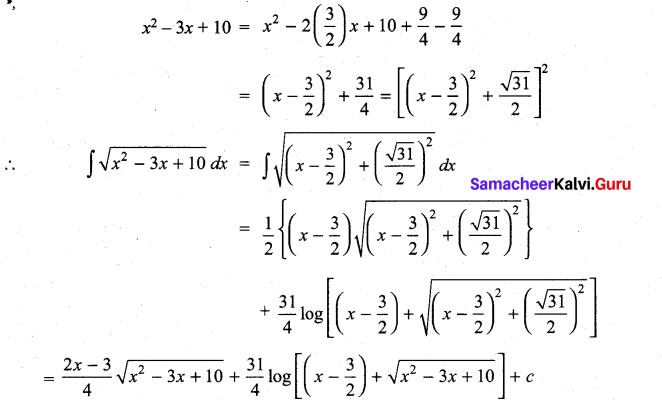Question 4.
$$\sqrt{169-(3 x+1)^{2}}$$
Solution: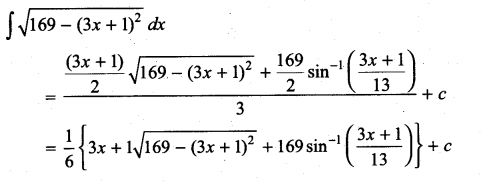Question 5.
$$\sqrt{1-3 x-x^{2}}$$
Solution: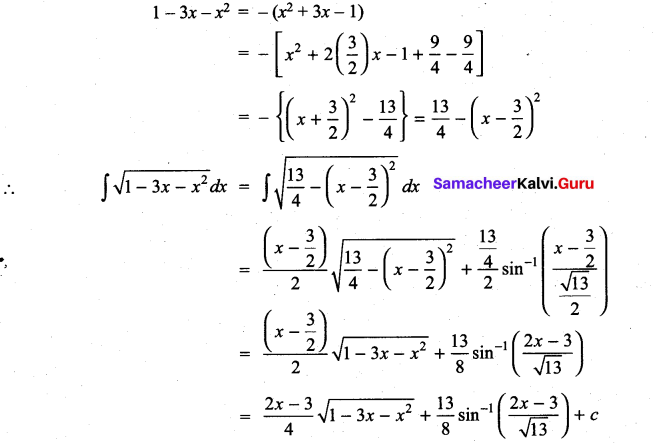Question 6.
$$\sqrt{(2-x)(3+x)}$$
Solution: